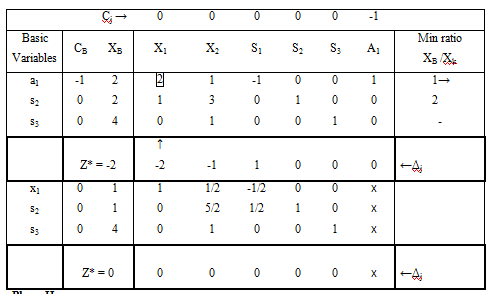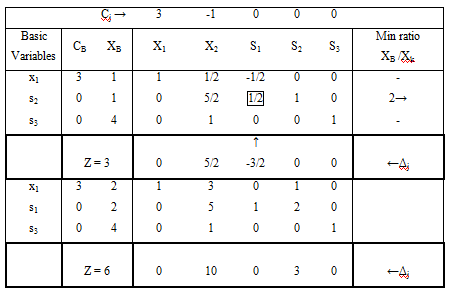#### Steps for Two-Phase Method, LPP Solutions

Steps for Two-Phase Method

The procedure of removing artificial variables is achieved in phase-I of the solution and phase-II is required to get an optimal solution. As the solution of LPP is calculated in two phases, it is known as Two-Phase Simplex Method.

Phase I - In this particular phase, the simplex method is applied to a exclusively constructed auxiliary linear programming problem leading to a final simplex table consisting a basic feasible solution to the original problem.

Step 1 - Allot a cost -1 to each artificial variable and a cost 0 to all the other variables in the objective function.

Step 2 - Make the Auxiliary LPP in which the new objective function Z* is to be maximized subject to the specified set of constraints.

Step 3 - Work out the auxiliary problem hrough simplex method until either of the following three possibilities do occur

i.      Max Z* < 0 and at least one artificial vector seems in the optimum basis at a positive level (Δj ≥ 0). In this case, given problem does not have any feasible solution.

ii.      Max Z* = 0 and at least one artificial vector seems in the optimum basis at a zero level. In this case one needs to proceed to phase-II.

iii.      Max Z* = 0 and no one artificial vector seems in the optimum basis. In this case one also needs to proceed for phase-II.

Phase II - Now allocate the actual cost to the variables in the objective function and a zero cost to each artificial variable that seems in the basis at the zero level. This new objective function is at present maximized by simplex method subject to the given constraints.

Simplex method is practically applied to the modified simplex table achieved at the end of phase-I, until an optimum basic feasible solution has been reached. The artificial variables which are non-basic at the finish of phase-I are removed.

Worked Examples

Example 1

Max Z = 3x1 - x2

Subject to

2x1 + x2 ≥ 2

x1 + 3x2 ≤ 2

x2 ≤ 4

&     x1 ≥ 0, x≥ 0

Standard LPP

Max Z = 3x1 - x2

Subject to

2x1 + x2 - s1+ a1= 2

x1 + 3x2 + s2  = 2

x2 + s3 = 4

x1 , x2 , s1, s2, s3,a≥ 0

Auxiliary LPP

Max Z* = 0x1 - 0x2 + 0s1 + 0s2 + 0s3 -1a1

Subject to

2x1 + x2 - s1+ a1= 2

x1 + 3x2 + s2  = 2

x2 + s3 = 4

x1 , x2 , s1, s2, s3,a≥ 0

Phase IIt is observed that all Δ≥ 0, Max Z* = 0 and no artificial vector seems in the basis, we move to phase II.

Phase IIBecause all Δj ≥ 0, optimal basic feasible solution is achieved, Thus the solution is Max Z = 6, x1 = 2, x2 = 0